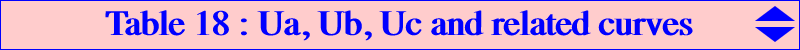These points Ua, Ub, Uc are defined in Special Isocubics §6.5. They are the only points for which there are infinitely many equilateral pKs with pivot one of these points. They lie on a large number of curves although they are not constructible with ruler and compass only. See also CL052 and Table 41.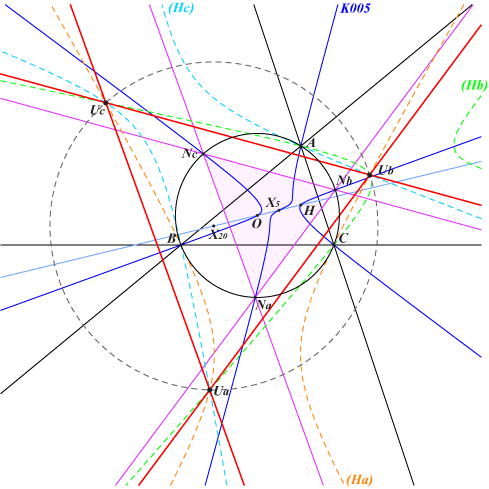Ua, Ub, Uc are the reflections of the orthocenter H about the points Na, Nb, Nc which are the intersections (apart A, B, C) of the Napoleon cubic K005 and the circumcircle of ABC. In other words, the triangle UaUbUc is the homothetic of NaNbNc under the homothety with center H and ratio 2. *** Let M be a point different of O and let M* be its isogonal conjugate. The vectors MM* and OH are equal if and only if M is one of the points Ua, Ub, Uc. *** These points Ua, Ub, Uc and their isogonal conjugates Ua*, Ub*, Uc* lie on the Neuberg cubic K001 and also on K732 which are two isogonal cubics. See also the associated points Va, Vb, Vc and Va*, Vb*, Vc* on the Neuberg cubic. At the very bottom of this page, a table gathers all the related cubics together.Conics Three hyperbolas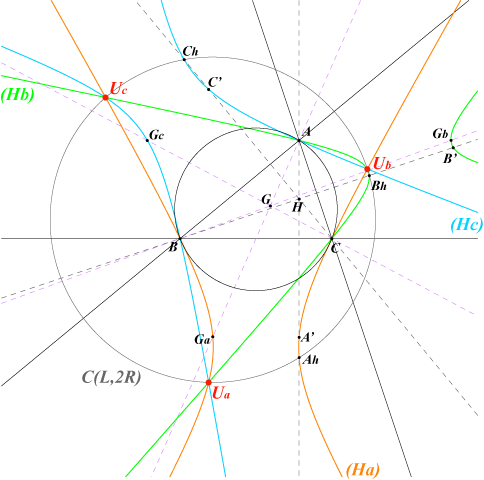Ga, Gb, Gc are the vertices of the antimedial triangle. Denote by (Ha) the hyperbola passing through B, C, Ga, the reflection A' of A in the line BC, the reflection Ah of H with respect to the second intersection of the altitude AH with the circumcircle. The asymptotes of (Ha) make 60° angles with the sideline BC hence (Ha) is a hyperbola with eccentricity 2. The center Oa is the midpoint of GaAh, a point on the perpendicular bisector of BC. (Hb) and (Hc) are defined similarly. These hyperbolas generate a net of conics passing through three points Ua, Ub, Uc.Other conics of the net Every conic of the net has the form : p (Ha) + q (Hb) + r (Hc) where P = (p:q:r) is any point in the plane. Obviously, P = A, B, C give the three hyperbolas. The net contains : • only one circle with P = X(69). This is the circle C(L,2R) where L = X(20) is the de Longchamps point. • a pencil of rectangular hyperbolas obtained when P lies on the de Longchamps line (trilinear polar of X(76), isotomic transform of the circumcircle). These rectangular hyperbolas always pass through O and are centered on the circle C(X(550), R) whereX(550) is the reflection of X(5) in O. The most remarkable are obtained when : P = X(850) passing through X(20), X(399), X(1147), X(2574), X(2575) P = X(523) passing through X(616), X(617) • a family of parabolas obtained when P lies on an ellipse centered at the intersection of the lines GK and X(30)-X(76). This ellipse does not contain any ETC center up to X(3053). When P is inside, outside this ellipse, the conic of the net is an ellipse, a hyperbola respectively. • a family of decomposed conics when P lies on the reunion of three lines forming a triangle circumscribing the ellipse above.Cubics Circum-cubics obtained with cevian lines Let P = p:q:r be a point and (La), (Lb), (Lc) its cevian lines. Any cubic of the form (La) (Ha) + (Lb) (Hb) + (Lc) (Hc) is a circum-cubic passing through P and the points Ua, Ub, Uc. All these cubics form a net which contains : one circular cubic K330 obtained with P = X(187). one equilateral cubic K092 obtained with P = G. a pencil of K0 cubics obtained when P lies on the Euler line. a pencil of cubics passing through O when P lies on the Brocard line. a pencil of cubics passing through G when P lies on the line G-X(187). *** Circum-cubics obtained with anticevian lines Let P = p:q:r be a point and (La), (Lb), (Lc) the sidelines of the anticevian triangle of P. Any cubic of the form (La) (Ha) + (Lb) (Hb) + (Lc) (Hc) is a circum-cubic passing through and the points Ua, Ub, Uc. All these cubics form a net which contains : one circular cubic K331 obtained when P = 1 / (a^4+b^4+c^4-3b^2c^2) : : , intersection of the lines {6, 620}, {25, 1634}, {111, 325}, etc. P is the isotomic conjugate of X(14568). one equilateral cubic K142 obtained with P = G. a family of K0 cubics obtained when P lies on a conic passing through X(892). *** Equilateral circum-cubics circumscribing the antimedial triangle K092 and K142 generate a pencil of equilateral circum-cubics passing through the points Ua, Ub, Uc and the vertices Ga, Gb, Gc of the antimedial triangle. This pencil contains K080 = KO++, the only K+. The equilateral triangle formed by the asymptotes has always center O. *** Circular circum-cubics through O K330 and K331 generate a pencil of circular circum-cubics passing through the points Ua, Ub, Uc and O. This pencil contains : one K0 which is the Neuberg cubic K001. three nK whose singular foci are the antipodes of A, B, C on the circum-circle and whose asymptotes are parallel to the sidelines of ABC. See figure 1 below. three focal cubics whose singular foci are A, B, C and whose asymptotes are the perpendicular at X(1657) to the sidelines of ABC. See figure 2 below.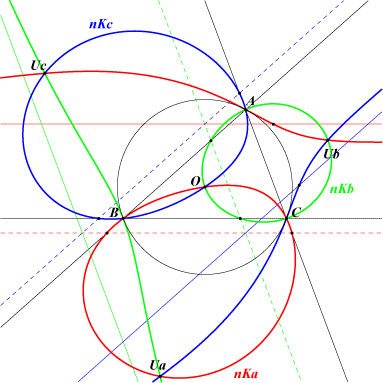Figure 1 : nK cubics nKa has singular focus the reflection Ao of A in O. The reflection of O in Ao lies on the curve. nKa is tangent at A to the circumcircle. It meets the sidelines AB, AC at two points lying on a parallel to BC. Its pole lies on AK and its root on AG.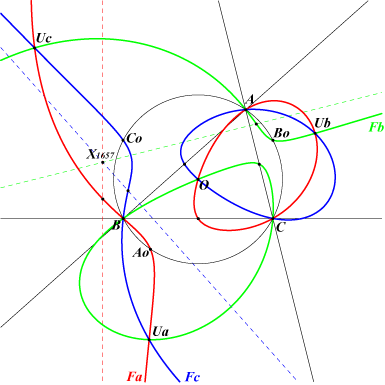Figure 2 : focal cubics All three cubics Fa, Fb, Fc pass through O. Fa has singular focus the point A. The reflection Ao of A in O lies on the curve. Fa contains the midpoint of BC and Ga, the A-vertex of the antimedial triangle. Its real asymptote is the perpendicular to BC at X(1657), the reflection of O in L.*** Cubics passing through O and H K001 and K080 generate a pencil of circum-cubics passing through the points Ua, Ub, Uc, O and H. This pencil contains the Lemoine cubic K009. See table 16 : the Neuberg-Lemoine pencil.Summary of points on cubic curvescubic Ua, Ub, Uc Ua*, Ub*, Uc* Va, Vb, Vc Va*, Vb*, Vc* nature of the cubic K001 • • • • circular isogonal pK K009 • CircumNormal nodal psK, spK K028 • stelloidal psK, spK K080 • stelloidal central spK K092 • equilateral isotomic pK K142 • equilateral K258 • nodal stelloid K330 • circular K331 • circular K405 • CircumNormal cubic K488 • K732 • • isogonal nK K753 • circular K902 • CircumTangential cubic K914 • circular cubic K922 • pK K923 •Two circum-cubics in cells of the same color are isogonal transformed from each other. K001 and K732 are self-isogonal cubics.Other curves These points Ua, Ub, Uc lie on : Q050 a circular quintic through G, O, H and the Fermat points Q051 a circular quartic through G and the Fermat points Q052 a circular quartic through G, O Q163 a quartic